International
Tables for
Crystallography
Volume D
Physical properties of crystals
Edited by A. Authier

International Tables for Crystallography (2006). Vol. D, ch. 1.8, pp. 224-226

## Section 1.8.4. Thermal conductivity

G. D. Mahana*

aDepartment of Physics, 104 Davey Laboratory, Pennsylvania State University, University Park, Pennsylvania, USA
Correspondence e-mail: gmahan@psu.edu

### 1.8.4. Thermal conductivity

| top | pdf |

#### 1.8.4.1. Introduction

| top | pdf |

The thermal conductivity determines the ability of the crystal to conduct heat. Device applications of crystals usually need an extreme value of the thermal conductivity: some applications need a low thermal conductivity, while others need a high thermal conductivity. At room temperature, the largest value of thermal conductivity is for diamond, which has K = 1.8 kW m−1 K−1; see e.g. Spitzer (1970), Slack (1979) or Berman (1976). The lowest values are for amorphous materials, which have K = 0.1 W m−1 K−1.

Heat flow can be carried by two kinds of excitations: phonons and electrons. The phonons carry most of the heat in insulators and semiconductors. Electrons carry appreciable amounts of heat only if there is a high density of conduction electrons, as in a metal. In metals, the electrons usually carry most of the heat. Of course, the heat conduction by phonons and electrons depends upon temperature. At high temperatures, the standard assumption is that the heat flows from phonons and electrons are independent and can be calculated separately. However, there is an electron–phonon interaction, which causes a correlation between the two kinds of heat flow. This is called phonon drag and is an important phenomenon at low temperatures – typically less than 50 K. We are concerned mostly with higher temperatures, so will not discuss phonon drag here.

First consider the heat flow carried by phonons. As a rule of thumb, crystals with high values of thermal conductivity are those that are: (1) tetrahedrally bonded, (2) contain elements of low atomic number, and (3) lack impurities and defects (Spitzer, 1970; Berman, 1976; Slack, 1979).

The inverse of the thermal conductivity is called the thermal resistivity. There is an equivalent of Matthiessen's rule for thermal conductivity: it is a reasonable approximation to take the various contributions to the thermal resistivity and simply to add them. This is not a rigorous theorem; it is just a process that gives a reasonable answer most of the time. Here we shall discuss four contributions to the thermal resistivity: boundary scattering, impurity scattering, isotope scattering and anharmonic inter­actions: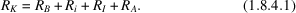These various terms are discussed in order.

#### 1.8.4.2. Boundary scattering

| top | pdf |

At low temperatures, the phonons that are thermally excited are those which have an energy near to or less than the thermal energy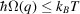. This usually means acoustic modes of long wavelength. They tend to have a long mean free path, which can extend to the size of the crystal. In this case, the limiting process on the phonon scattering is simply bouncing off the walls of the crystal. The formula for this process is best derived from the classical formula for the thermal conductivity (see Ziman, 1962),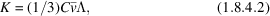where C is the heat capacity,is the average velocity andis the mean free path. To apply this to the present problem, taketo be equal to the average dimension of the crystal andto be the speed of sound averaged over the various directions. At low temperatures, the heat capacity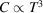, as given by the Debye theory. Sinceandare constants, then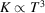, which agrees well with the dependence found experimentally. Of course, the thermal resistance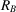is just the inverse of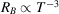.

#### 1.8.4.3. Impurity scattering

| top | pdf |

Impurities can be either point defects or extended defects such as dislocations. Here we confine our remarks to point defects. When acoustic phonons of long wavelength scatter from point defects, the process is very much like the Rayleigh scattering of light. The basic cross section varies as the fourth power of the frequency. Equivalently, at long wavelength, it varies as the fourth power of the wave number q of the phonons (see Ziman, 1962):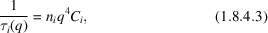whereis the concentration of impurities andis a constant characteristic of the impurity. Of course, this lifetime for the phonon of wave number q must be averaged over all of the wavevectors in the crystal as a function of temperature. This averaging is actually mathematically delicate. At temperatures T less than the Debye temperature, the average value of q is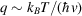and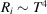. At high temperatures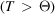, the average of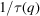is a constant, since all values of q in the Brillouin zone are equally accessible. In this limit,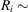constant.

#### 1.8.4.4. Isotope scattering

| top | pdf |

The perfect crystal is defined as having each atom in its expected position, with no vacancies, interstitials or other defects. Such a crystal would still have a type of disorder that scatters phonons. Most elements have several natural isotopes for their nuclei. Natural crystals usually reflect this mixture of isotopes. Special crystals can be made that are composed of a single isotope, and these lack the resistive term from isotope scattering. However, most crystals have isotope scattering. The scattering is from the mass difference of the nuclei. The interaction term comes from the kinetic energy of the ion vibrational motion. If there are two isotopes with massesand, with concentrations c and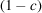, respectively, then the average of the mass in the kinetic energy term as discussed by Klemens (1955) is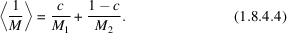The kinetic energy term of the ions is then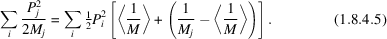The second term is the perturbation. When we evaluate the golden rule for scattering, we square the matrix element and then average the square: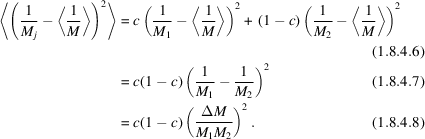The isotope scattering depends upon the concentration in the form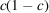and upon the square of the mass difference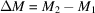. The isotope fluctuations act as point defects. The total expression for the scattering cross section also includes a factor of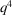in addition to the factors given above. Thus their temperature dependence is identical to that of the point defects: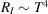at low temperature and RI ~ constant at high temperature.

This behaviour is found experimentally. The isotope scattering is usually a small contribution to the thermal resistivity. It is only important in temperature regions where the other resistivities are small. This occurs, of course, at the maximum value of the thermal conductivity, since that is where all of the resistivities are small. Changing the isotopic mix of a crystal changes the thermal conductivity in the temperature regions where it is large. One example is diamond, which is usually 99% 12C and 1% 13C. Anthony et al. (1990) showed that eliminating the 13C increases the thermal conductivity by a factor of nearly two (1.8 to 3.2 kW m−1 K−1) at room temperature. Another example is germanium, where isotope scattering makes a sizeable contribution to the thermal resistance (see Berman, 1976).

#### 1.8.4.5. Alloy scattering

| top | pdf |

Alloys are mixtures of two or more different crystal `ingredients'. We assume that the atoms are randomly located on the different atom sites. Some alloys are ordered, but that makes them crystals. An example of a disordered alloy is GaxAl1−xAs. Since GaAs and AlAs have the same crystal structure and nearly the same lattice constant, the mixed crystal permits any value of x. The Ga and Al atoms randomly occupy the cation site in the zinc blende lattice. Experimentally, it is found that the thermal resistance as a function of x is (see Ziman, 1962; Berman, 1976)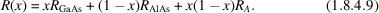The first two terms just average the thermal resistance of the two lattices. The third term is the scattering of the phonons from the alloy fluctuations. It is derived in the same way as the equivalent factor ofin the discussion of isotope scattering in Section 1.8.4.4. In alloys, the fluctuations are due to two factors: the mass difference at the atoms sites (as in isotope scattering) and the difference in the bonding between Ga and Al. The constant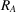depends on these factors. It is not small: Yao (1987) showed that the term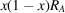is four or five times larger than the others at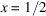. Although we have cited a particular example of alloy scattering, this dependence is quite universal. Alloy fluctuations typically dominate the thermal resistivity of alloys.

#### 1.8.4.6. Anharmonic interactions

| top | pdf |

In crystals that are relatively pure, i.e. those that lack large numbers of impurities, the important limitation on thermal conductivity at high temperature is from anharmonic interactions (Ziman, 1962). The vibrational potential between neighbouring atoms is not perfectly harmonic. Besides the quadratic dependence on vibrational distance, there is usually a term that depends upon the third and perhaps fourth powers of the relative displacements of the ions. These latter terms are the anharmonic part of the vibrational potential energy. They cause the crystal to expand with temperature and also contribute to the thermal resistance.

For most crystals, the cubic term is important. Its contribution is best explained using the language of phonons. The cubic term means that three phonons are involved. This usually means that one phonon decays into two others, or two phonons combine into one. Both processes contribute to the lifetime of the phonons. On rare occasions, the phase space of the phonons does not permit these events. For example, silicon has a very high frequency optical phonon branch (62 meV at the zone centre) while the acoustic phonons have rather low frequencies. The optical phonons are unable to decay into two of lower frequency, since the two do not have enough energy. This explains, in part, why silicon has a high thermal conductivity. However, this case is unusual. In most crystals, the phonons have similar energy and one can decay into two of lower energy.

The three-phonon events have a simple dependence upon temperature. When one phonon goes to two, or vice versa, the rate depends upon the density of phonons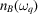as given by the Bose–Einstein occupation number. At high temperature, i.e. about half of the Debye temperature, this function can be expanded to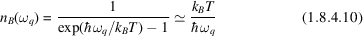and the thermal resistance is proportional to temperature. Thus a plot of the inverse thermal conductivity versus temperature usually shows a linear behaviour at high temperature. This linear term is from the anharmonic interactions. There are two main reasons for deviations from linear behaviour: the thermal expansion of the crystal and the contribution of the anharmonic quartic terms, which tend to go as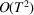.

#### 1.8.4.7. Thermal conductivity of metals

| top | pdf |

Heat conduction in metals can occur by either phonons or electrons. The conduction by phonons has been discussed above. In metals, there is another contribution to the thermal resistance: the absorption of the phonons by the electrons. Metals have low-energy excitations, which consist of exciting an electron just below the chemical potential to an occupied state just above the chemical potential. The separation in energy between these two electron states can be arbitrarily small and can be small enough to be equal to the energy of a phonon. By this process, the energy of the phonon can be absorbed by the electron gas, which contributes to the thermal resistance of the phonons.

However, in metals, the electrons tend to carry more heat than the phonons. The latter play a secondary role. Thus, we divide the thermal conductivity into electronic and phonon parts,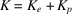. This choice of separation is rather interesting. Note that we do not combine their inverses, as we do for the components of each separate contribution.

The thermal resistance due to the electrons is related to the electrical resistance. Both depend upon the lifetime of the electrons. Because of this, there is a simple relationship between the electronic part of the thermal conductivity and the electrical conductivity. This relationship is called the Wiedemann–Franz law (Wiedemann & Franz, 1853).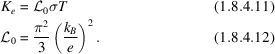The parameter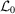is called the Lorenz number. The value given above is for a metal with a well defined Fermi surface, so the electrons obey Fermi–Dirac statistics. In the other limit of classical statistics, its value is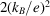. We caution that this simple relation between the electrical and thermal conductivities is not exact. The reason for this is that the two lifetimes are not identical: the electrical conductivity uses the lifetime for changing the momentum of the electron, while the thermal conductivity uses the lifetime for changing the energy current. However, the two lifetimes are similar. In practice, the Wiedemann–Franz law is found to work quite well. It seems to be valid regardless of the mechanisms that scatter the electrons: whether the scattering is by phonons, impurities or spin excitations. It can be used to estimate the thermal conductivity from electrons in metals, or in semiconductors with large densities of conduction electrons or holes.

### References

Anthony, T. R., Banholzer, W. F., Fleischer, J. F., Wei, L., Kuo, P. K., Thomas, R. L. & Pryor, R. W. (1990). Thermal diffusivity of isotropically enriched 12C diamond. Phys. Rev. B, 42, 1104–1111.
Berman, R. (1976). Thermal conduction in solids. Oxford University Press.
Klemens, P. G. (1955). The scattering of low-frequency lattice waves by static imperfections. Proc. Phys. Soc. London Sect. A, 68, 1113–1128.
Slack, G. A. (1979). The thermal conductivity of nonmetallic crystals. In Solid state physics, Vol. 34, edited by H. Ehrenreich, F. Seitz & D. Turnbull, pp. 1–71. New York: Academic Press.
Spitzer, D. P. (1970). Lattice thermal conductivity of semiconductors: a chemical bond approach. J. Phys. Chem. Solids, 31, 19–40.
Wiedemann, G. & Franz, R. (1853). Ann. Phys. (Leipzig), 89, 497.
Yao, T. (1987). Thermal properties of AlAs/GaAs superlattices. Appl. Phys. Lett. 51, 1798–1782.
Ziman, J. M. (1962). Electrons and phonons. Oxford University Press.